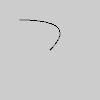# Py5Shape.bezier_vertex()#

Specifies a Py5Shape object’s vertex coordinates for Bezier curves.

## Examples#def setup():
py5.size(100, 100, py5.P2D)
s = py5.create_shape()
s.begin_shape()
s.no_fill()
s.vertex(30, 20)
s.bezier_vertex(80, 0, 80, 75, 30, 75)
s.end_shape()
py5.shape(s)def setup():
py5.size(100, 100, py5.P2D)
s = py5.create_shape()
s.begin_shape()
s.vertex(30, 20)
s.bezier_vertex(80, 0, 80, 75, 30, 75)
s.bezier_vertex(50, 80, 60, 25, 30, 20)
s.end_shape()
py5.shape(s)


## Description#

Specifies a Py5Shape object’s vertex coordinates for Bezier curves. Each call to bezier_vertex() defines the position of two control points and one anchor point of a Bezier curve, adding a new segment to a line or shape. The first time bezier_vertex() is used within a Py5Shape.begin_shape() call, it must be prefaced with a call to Py5Shape.vertex() to set the first anchor point. This method must be used between Py5Shape.begin_shape() and Py5Shape.end_shape() and only when there is no MODE parameter specified to Py5Shape.begin_shape().

Drawing 2D bezier curves requires using the P2D renderer and drawing 3D bezier curves requires using the P3D renderer. When drawing directly with Py5Shape objects, bezier curves do not work at all using the default renderer.

Underlying Processing method: PShape.bezierVertex

## Signatures#

bezier_vertex(
x2: float,  # the x-coordinate of the 1st control point
y2: float,  # the y-coordinate of the 1st control point
x3: float,  # the x-coordinate of the 2nd control point
y3: float,  # the y-coordinate of the 2nd control point
x4: float,  # the x-coordinate of the anchor point
y4: float,  # the y-coordinate of the anchor point
/,
) -> None

bezier_vertex(
x2: float,  # the x-coordinate of the 1st control point
y2: float,  # the y-coordinate of the 1st control point
z2: float,  # the z-coordinate of the 1st control point
x3: float,  # the x-coordinate of the 2nd control point
y3: float,  # the y-coordinate of the 2nd control point
z3: float,  # the z-coordinate of the 2nd control point
x4: float,  # the x-coordinate of the anchor point
y4: float,  # the y-coordinate of the anchor point
z4: float,  # the z-coordinate of the anchor point
/,
) -> None


Updated on March 06, 2023 02:49:26am UTC